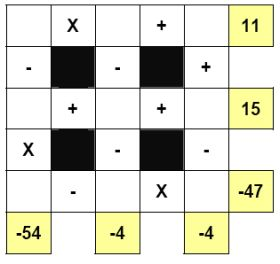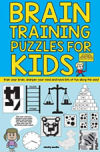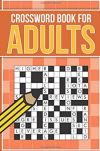Thu, 09 Jul 2020

We can provide a range of maths games and puzzles, aimed at both children and adults. Popular maths puzzles include number sequences, written puzzles that require the formation of mathematical equations and varying sophistication to be solved, and maths square puzzles.

One of our most popular maths related puzzles is the so-called Math Square.Each number from 1 - 9 is placed once per puzzle; the aim is to work out where each number goes to solve the equations. You can view this Maths Square Puzzle in PDF format complete with solution.

There are two versions of this puzzle available. The first, and more common in publication, requires you to perform the sums from left to right and top to bottom. The second less common version requires the calculations to be performed in strict BODMAS order, so for instance you perform multiplications before subtraction or additions... this is the case in the sample puzzle shown here and the sample PDF also.

The maths square comes in a range of sizes from 3 x 3 which is perfect for younger audiences, right through to much larger sizes for a seriously difficult adult challenge!

Alternatively, take a look at some other word and non-word puzzles that Clarity Media can supply you with:
Puzzle Solutions
i Newspaper Solutions

Book Ideas: For ChildrenBrain Training for KidsView a single page overview
list of puzzles

list of kids puzzles

Word Puzzles
Children's Puzzles
Wordsearch
CodeWords
Arrow words
Kriss Kross
Word Wheel

Number & Colouring Puzzles
Children's Sudoku
Samurai Sudoku
Jigsaw Sudoku
Futoshiki
Mind Games
Killer Sudoku
Kakuro
Chess Puzzles
A - Z of Puzzles
Colour By Number
Dot To Dot

Puzzle Tools
Puzzle FAQ
Codeword Solver
Rhyming Dictionary
Puzzle Solving Tools

Services
Puzzles for Apps
Article Writing
Themed Puzzle Pages